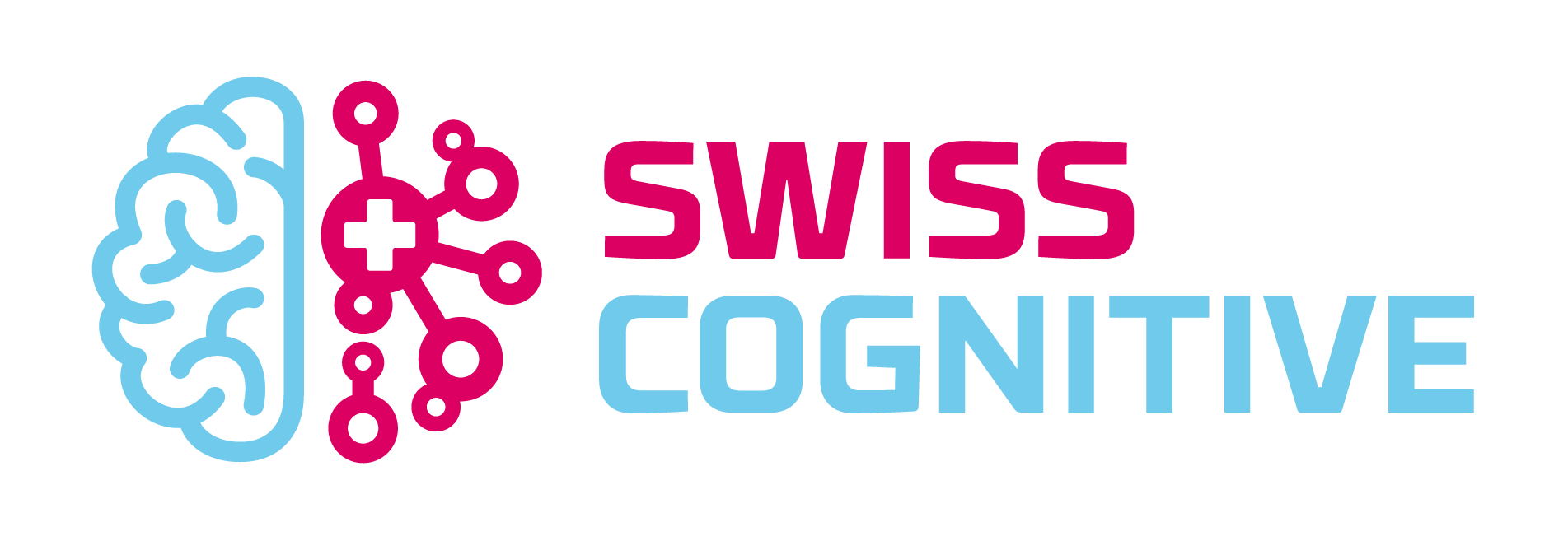## Deep learning can help discover mathematical relations that evade human scientists, a recent paper by researchers at DeepMind shows. Like many things coming from the Alphabet-owned artificial intelligence lab, the paper, which is titled “Advancing mathematics by guiding human intuition with AI,” has received much attention from science and tech media.Some mathematicians and computer scientists have lauded DeepMind’s efforts and the findings in the paper as breakthroughs. Others are more skeptical and believe that the use of deep learning in mathematics might have been overstated in the paper and its coverage in popular press.

This article is part of a reviews of AI research papers, a series of posts that explore the latest findings in artificial intelligence.

The results are nonetheless fascinating and can expand the toolbox of scientists in discovering and proving mathematical theorems.

### A framework for mathematical discovery with machine learning

In their paper, the scientists at DeepMind suggest that AI can be used to “assist in the discovery of theorems and conjectures at the forefront of mathematical research.” They propose a “framework for augmenting the standard mathematician’s toolkit with powerful pattern recognition and interpretation methods from machine learning.”Mathematicians start by making a hypothesis about the relation between two mathematical objects. To verify the hypothesis, they use computer programs to generate data for both types of objects. Next, a supervised machine learning model algorithm crunches the numbers and tries to tune its parameters that map one type of object to the other.

“The key contributions of machine learning in this regression process are the broad set of possible nonlinear functions that can be learned given a sufficient amount of data,” the researchers write. […]